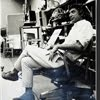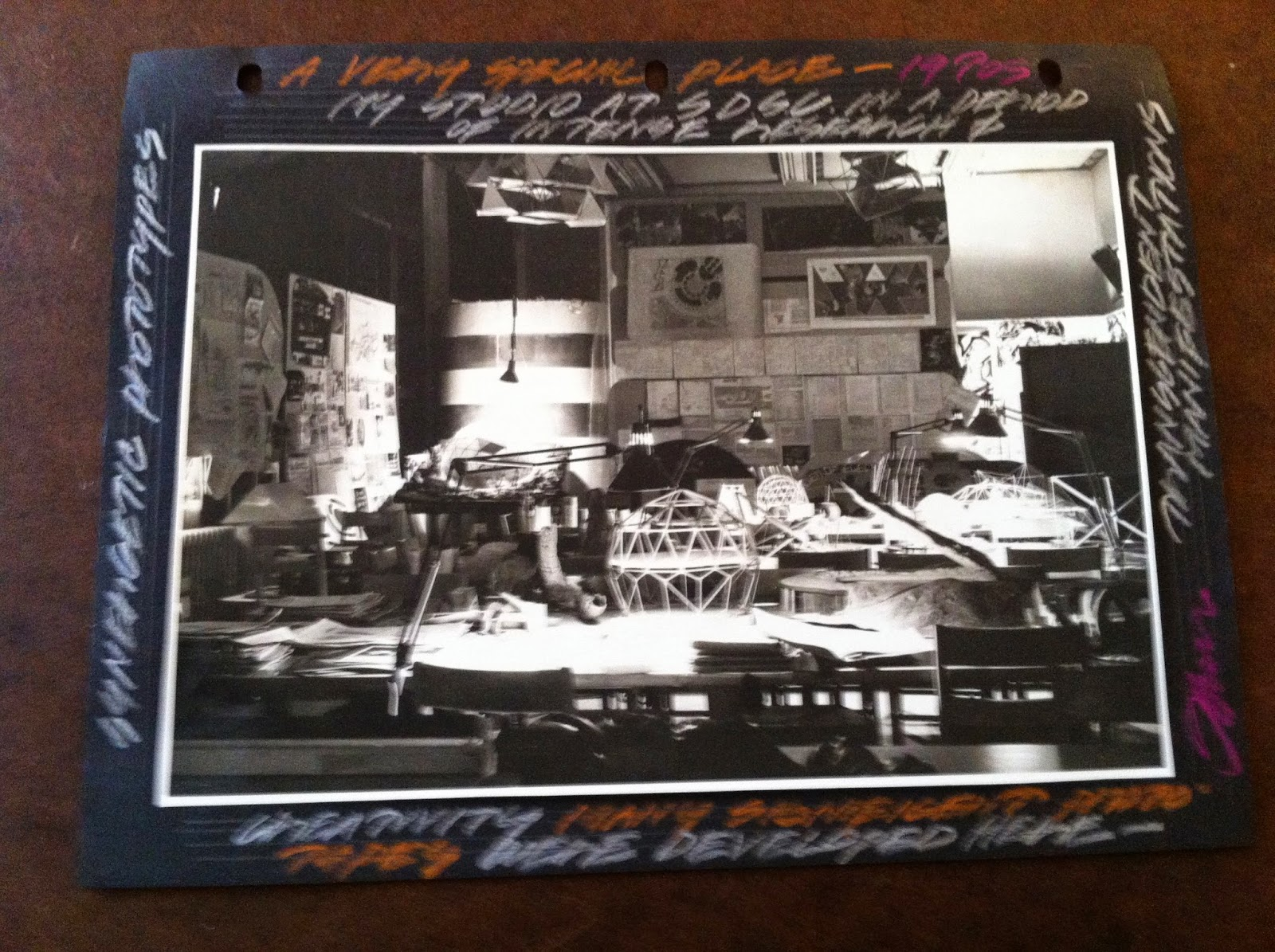## Eugene Ray SDSU 1970'sEugene Ray 1970's. photo credit: Tom Davis

## Saturday, May 31, 2014

### FLASH OF LIGHT / evening sky

+ARCHITECTURE IS NOT JUST MATTER+
>it is more importantly what you don't see that is<
>an essence that makes architecture so important<
+FULCANELLI+
>and other enlightened egyptian alchemist seers<
>(papus & peladan) at the marvelous bookshop<
^^^^^^^^^^^^^^^^^^^^^^^^^^^^^^
+GOOGLE : EUGENE RAY / DEGRUY/
+BOOKSHOP OF THE MARVELOUS+
^^^^^^^^^^^^^^^^^^^^^^^^^^^
>bookshops in paris in the 19th century<
>were magnificent gathering places for<
>scholars of alchemy who were great<
+ARTISTS & MAGICIANS /+
+ST SULPICE SCHOLARS+
>were on the cutting edge of a creative<
>exploration for an enlightened world<
^^^^^^^^^^^^^^^^^^^^^^
>notre dame cathedral (on the cover here)<
>was a gothic geomancy st bernard vision<
+(CORBU was not CATHOLIC,+
>that inspired scholars at st sulpice, such<
>as pere couturier corbu's great patron<
^^^^^^^^^^^^^^^^^^^^^
>stonehenge is one of those ancient cosmic<
>inspired sites that illuminated st bernard<
+(ET CROP CIRCLES+
+SEEN HERE (many)) !
>and his pere couturier scholar corbu<
^^^^^^^^^^^^^^^^^^^^^^
>de ray castle on the saone river in burgundy<
>is where the turin shroud was first brought<
+/ DEGRUY / de RAY CASTLE)+
>there were 13 original towers in the 12th C<
^^^^^^^^^^^^^^^^^^^^^^^^^^
>in 1965 this, probably the best biomorphic<
>design, i have ever made flowed from my<
+(COSMIC BIOTRONICS via+
+COMPOUND CURVES)+ !
>pencil as if by a stroke of synchronicity<
^^^^^^^^^^^^^^^^^^^^^^^
>four years later, by 1969 i had completed<
>four projects in new orleans of color, light<
+COLOR-LIGHT- ENERGY+
>and gathered my family to head to sdsu to<
> do my best to share the vision w/ others<
^^^^^^^^^^^^^^^^^^^^^^^^^^^
>(announcement for my retrospective<
>of 1980 at san diego state university)<
^^^^^^^^^^^^^^^^^^^^^^^^
>synchronistically by 1980 i had completed<
>"silver ship" and constructed an exhibition<
+10 YEARS IN CALIFORNIA)+
>of all the work in california for 10 years<
^^^^^^^^^^^^^^^^^^^^^^^^^^
>synchronistically "silver ship" was built on<
>nautilus st. (perfection) in lajolla california<
^^^^^^^^^^^^^^^^^^^^^^^^^
>the technology of ufo craft is mind boggling<
>only a study of our natural elements can be<
+(COMPOUND CURVED+
+UFOs PLUS SHELLs+
+ARE VERY STRONG)+ !
>comparative to the ufo structural perfection<
^^^^^^^^^^^^^^^^^^^^^^^^^^
>only leonardo da vinci was advanced enough<
>in the 16th century to design this fortress for<
+(COMPOUND CURVE WALLS+
+PROVIDE MUCH STRENGTH)+
>"valentine" borgia, francois I brother-in-law<
^^^^^^^^^^^^^^^^^^^^^^^^^^^^
>the dna memory phenomena is fascinating<
>and constantly links my focus with the<
+TO ST. BERNARD VISION+
>ray-bresse-gruy-savoy-vergy-charney<
^^^^^^^^^^^^^^^^^^^^^^^^>our sdsu classroom-studios were settings<
>for much technical vision & exploration<
^^^^^^^^^^^^^^^^^^^^^^^^^>little did i realize at the time how important<
>our effort at san diego state, tulane & lsu<
+(OUR MESSAGE IS NOW+
+SEEN DAILY in EUROPE)+ !
>would ultimately be via the world wide<
>link of this website to many countries<
^^^^^^^^^^^^^^^^^^^^^^^
>kerlian photography has revealed the energy<
>emitted from pyramids in several countries<
^^^^^^^^^^^^^^^^^^^^^^^^^
>tonight's blog ends w/ an image from the<
>crash at roswell on the cover of dr. bob<
>and ryan woods ufo crash conf. book<
+(1947 WAS the YEAR of MY+
+SIGHTING plus ROSWELL)+
>next to a page from ron edens book<
>devoted to my life & work which<
>provides a very interesting sync<
^^^^^^^^^^^^^^^^^^^^^
+GEORGE NOORY BELIEVES+
+there are NO COINCIDENCES+ !
^^^^^^^^^^^^^^^^^^^^^^
eugene ray, mfa, architect
professor emeritus, sdsu